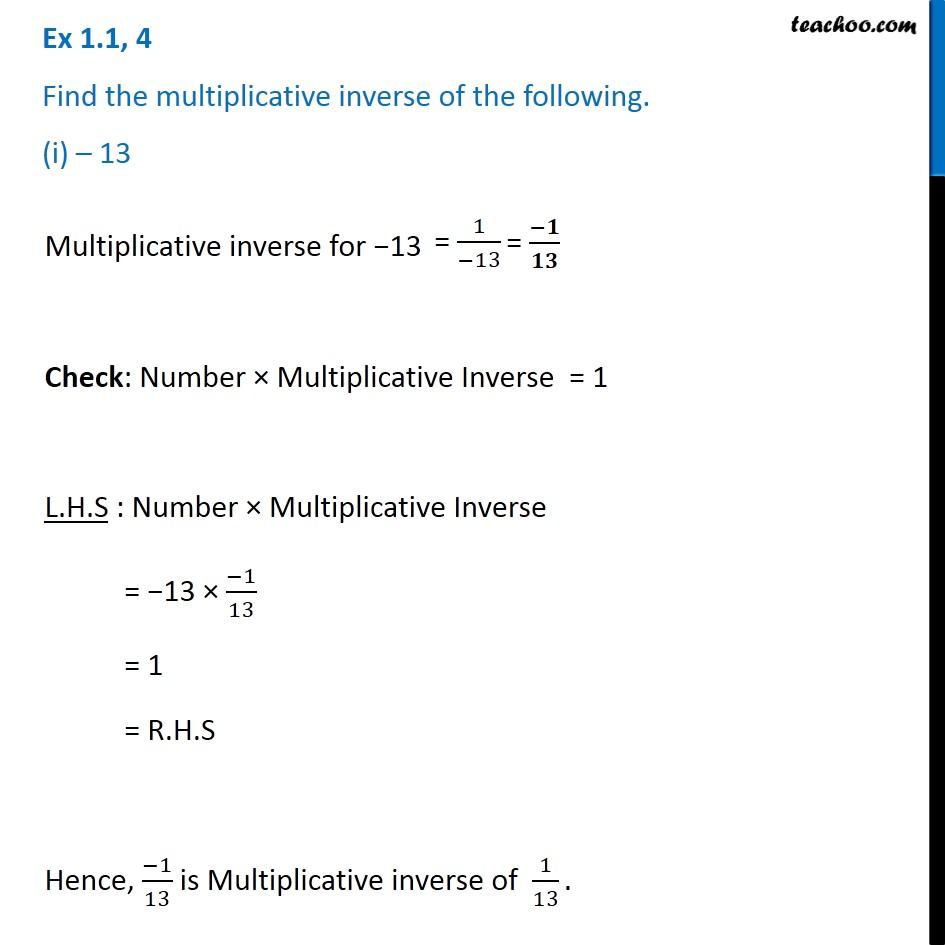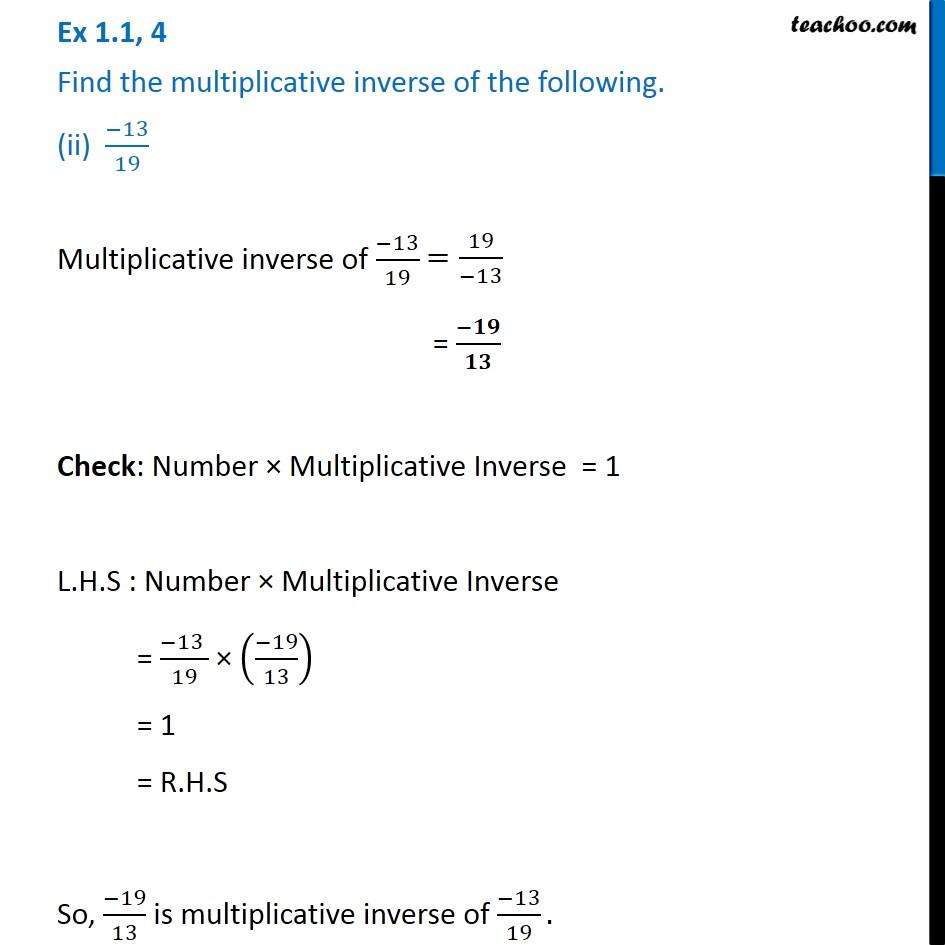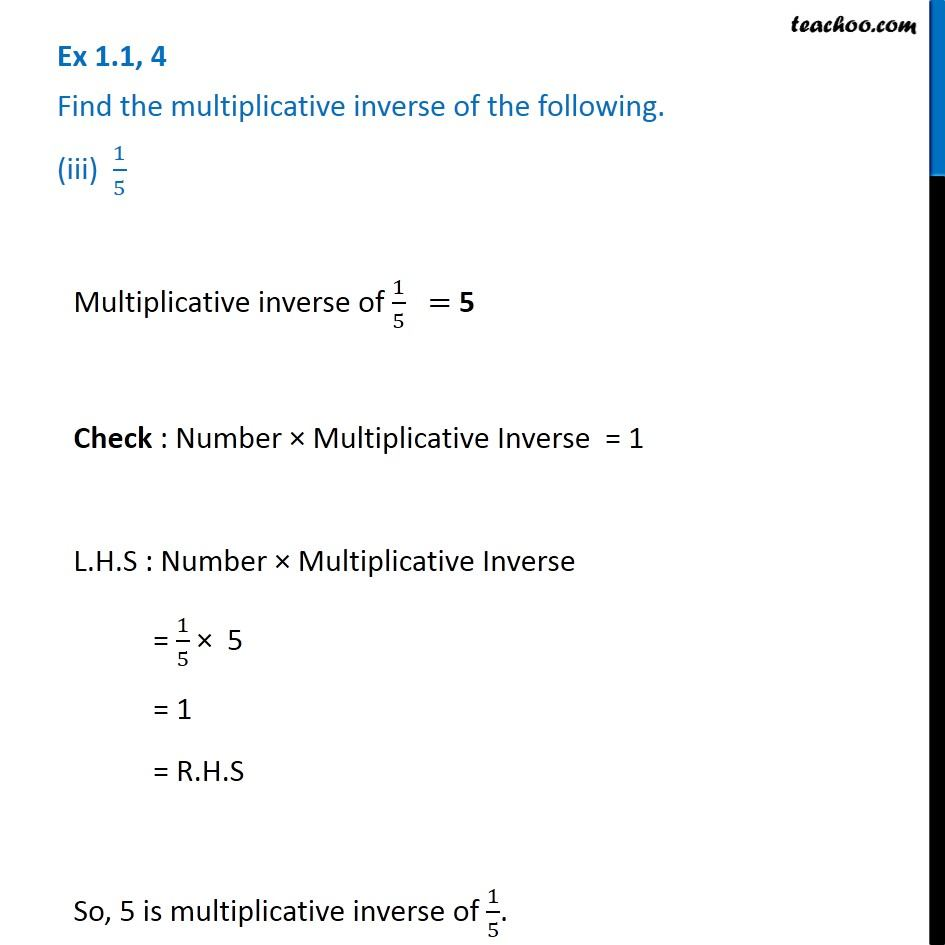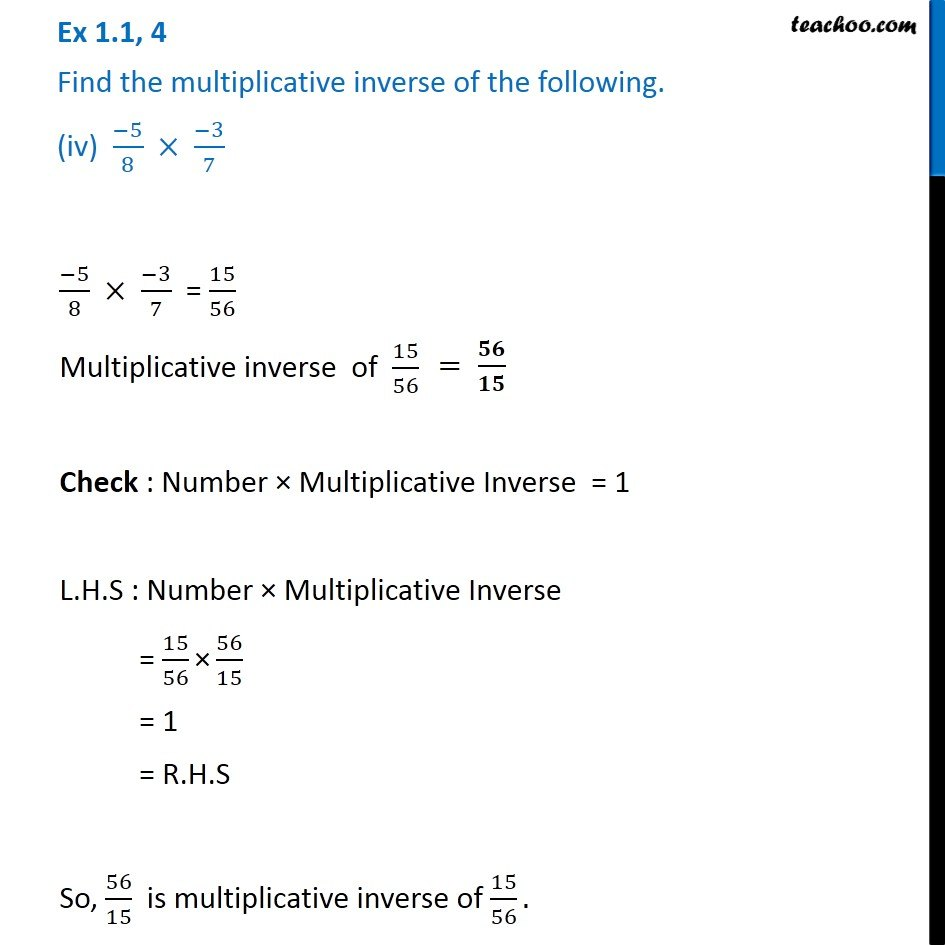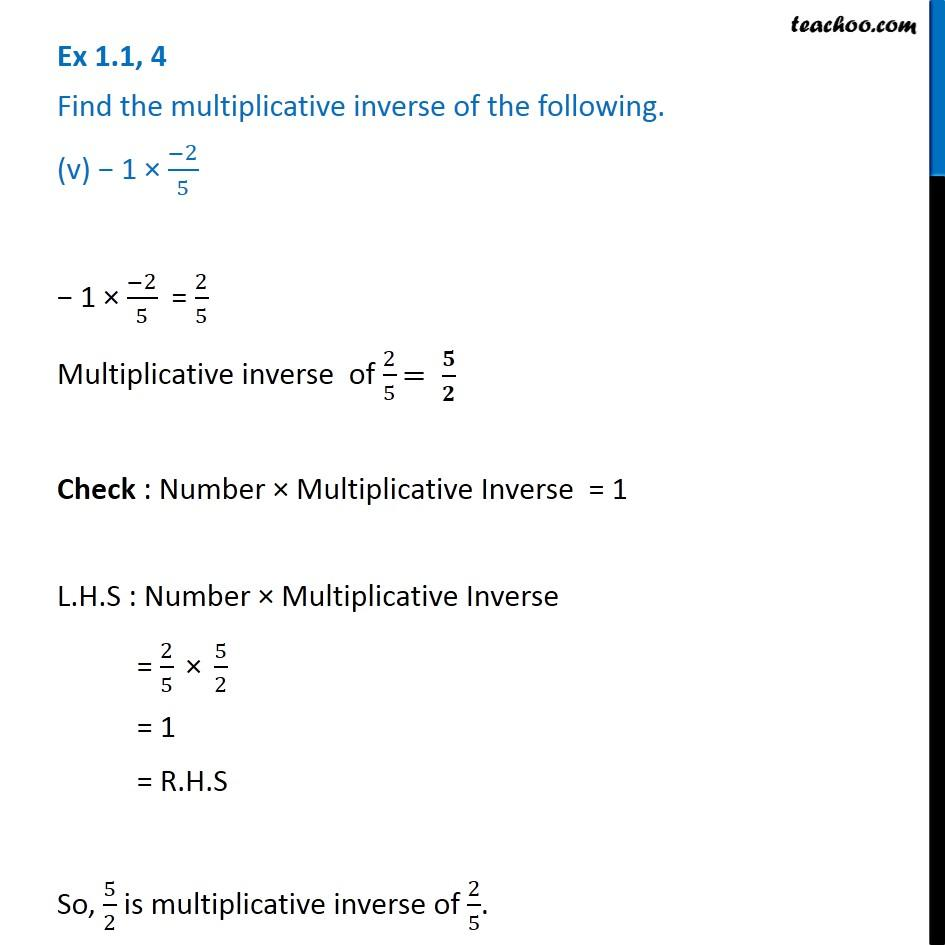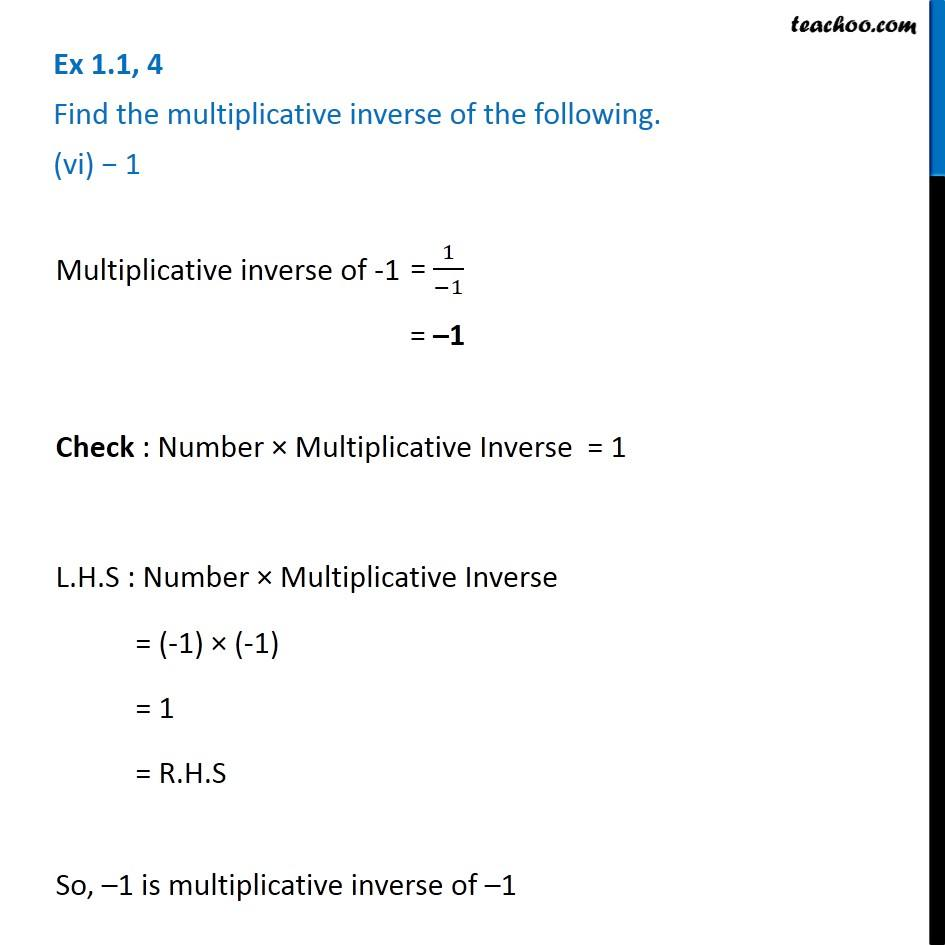Subscribe to our Youtube Channel - https://you.tube/teachoo

1. Chapter 1 Class 8 Rational Numbers
2. Serial order wise
3. Ex 1.1

Transcript

Ex 1.1, 4 Find the multiplicative inverse of the following. (i) 13 Multiplicative inverse for 13 Check: Number Multiplicative Inverse = 1 L.H.S : Number Multiplicative Inverse = 13 ( 1)/13 = 1 = R.H.S Hence, ( 1)/13 is Multiplicative inverse of 1/13. Ex 1.1, 4 Find the multiplicative inverse of the following. (ii) ( 13)/19 Multiplicative inverse of ( 13)/19 = ( )/ Check: Number Multiplicative Inverse = 1 L.H.S : Number Multiplicative Inverse = ( 13 )/19 (( 19)/13) = 1 = R.H.S So, ( 19)/13 is multiplicative inverse of ( 13)/19. Ex 1.1, 4 Find the multiplicative inverse of the following. (iii) 1/5 Multiplicative inverse of 1/5 Check : Number Multiplicative Inverse = 1 L.H.S : Number Multiplicative Inverse = 1/5 5 = 1 = R.H.S So, 5 is multiplicative inverse of 1/5. Ex 1.1, 4 Find the multiplicative inverse of the following. (iv) ( 5)/8 ( 3)/7 ( 5)/8 ( 3)/7 = 15/56 Multiplicative inverse of 15/56 Check : Number Multiplicative Inverse = 1 L.H.S : Number Multiplicative Inverse = 15/56 " " 56/15 = 1 = R.H.S So, 56/15 is multiplicative inverse of 15/56. Ex 1.1, 4 Find the multiplicative inverse of the following. (v) 1 ( 2)/5 1 ( 2)/5 = 2/5 Multiplicative inverse of 2/5 Check : Number Multiplicative Inverse = 1 L.H.S : Number Multiplicative Inverse = 2/5 " " 5/2 = 1 = R.H.S So, 5/2 is multiplicative inverse of 2/5. Ex 1.1, 4 Find the multiplicative inverse of the following. (vi) 1 Multiplicative inverse of -1 = 1 Check : Number Multiplicative Inverse = 1 L.H.S : Number Multiplicative Inverse = (-1) (-1) = 1 = R.H.S So, 1 is multiplicative inverse of 1

Ex 1.1